Published © CC BY-ND

# Using MATLAB to Implement a Distortion Effect on Zynq

Project where we will develop an overdrive guitar effect with MATLAB and Zynq.

IntermediateFull instructions provided4 hours55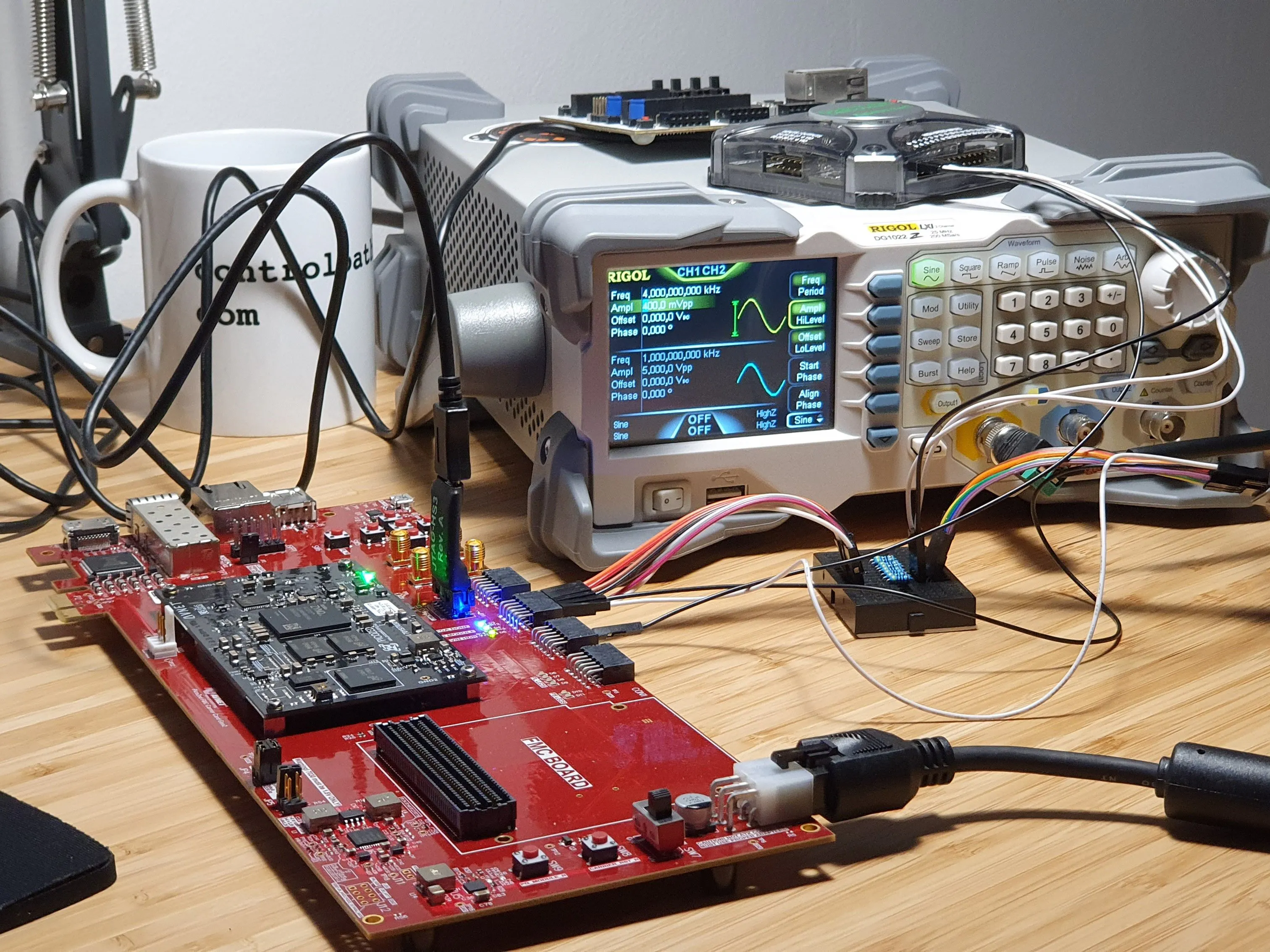## Things used in this project

### Hardware components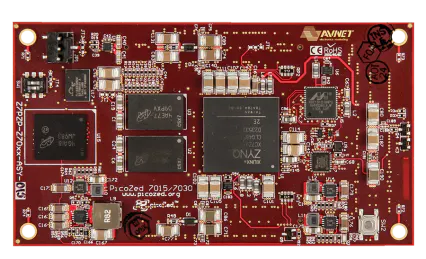Avnet PicoZed
×1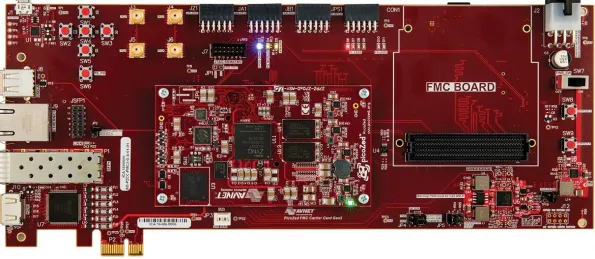Avnet PicoZed FMC Carrier Card V2
×1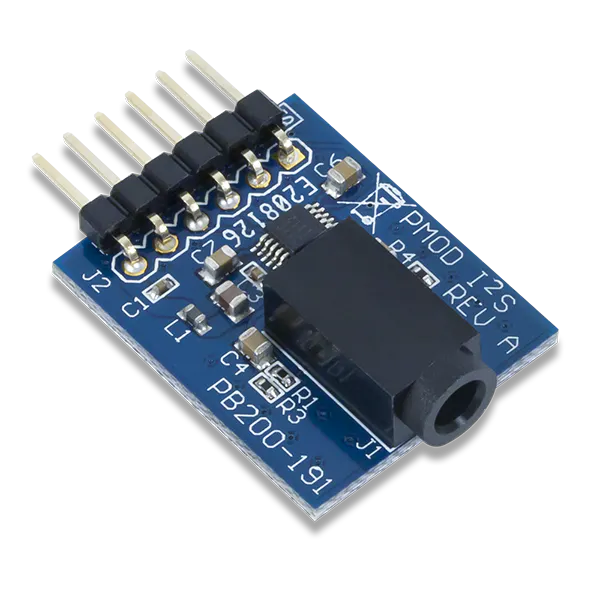Digilent Pmod I2S
×1

### Software apps and online services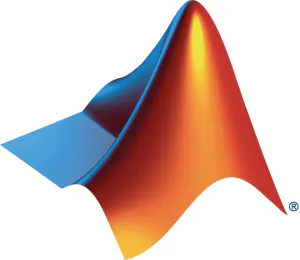MATLABXilinx Vivado Design Suite

Read more

## Code

### Code snippet #3

Plain text
```close all
clear all
clc

%% Fir taps compute

taps = fir1(4,0.000001)

%% AXI registers configuration
h = aximaster('Xilinx')

enable = 1;

% FIR configuration
b0 = taps(1);
b1 = taps(2);
b2 = taps(3);
b3 = taps(4);
b4 = taps(5);

% Convert to fixed point
b0_qq = floor(b0*2^22);
b1_qq = floor(b1*2^22);
b2_qq = floor(b2*2^22);
b3_qq = floor(b3*2^22);
b4_qq = floor(b4*2^22);

% write AXI registers
h.writememory('40010118',0)

h.writememory('40010104',b0_qq)
h.writememory('40010108',b1_qq)
h.writememory('4001011C',b2_qq)
h.writememory('4001010C',b3_qq)
h.writememory('40010110',b4_qq)

h.writememory('40010118',enable)

release(h);
```

### Code snippet #4

Plain text
```close all
clear all
clc

%% Fir taps compute

taps = fir1(4,0.000001)

%% AXI registers configuration
h = aximaster('Xilinx')

enable = 1;

% FIR configuration
b0 = taps(1);
b1 = taps(2);
b2 = taps(3);
b3 = taps(4);
b4 = taps(5);

% Convert to fixed point
b0_qq = floor(b0*2^22);
b1_qq = floor(b1*2^22);
b2_qq = floor(b2*2^22);
b3_qq = floor(b3*2^22);
b4_qq = floor(b4*2^22);

% write AXI registers
h.writememory('40010118',0)

h.writememory('40010104',b0_qq)
h.writememory('40010108',b1_qq)
h.writememory('4001011C',b2_qq)
h.writememory('4001010C',b3_qq)
h.writememory('40010110',b4_qq)

h.writememory('40010118',enable)

release(h);
```

### Github

https://github.com/Digilent/Pmod-I2S2

### Overdrive effect Matlab

Simulink models used on this project.

## Credits

### Pablo Trujillo

10 projects • 37 followers
FPGA designer for power electronics equipment. DSP and Power Electronics Control design specialist.